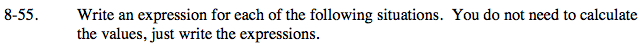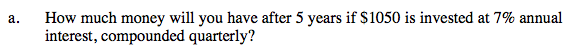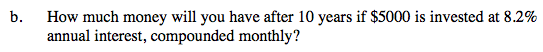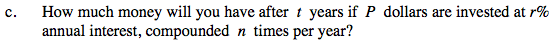### Home > PC > Chapter 8 > Lesson 8.1.4 > Problem8-55

8-55.
1. Write an expression for each of the following situations. You do not need to calculate the values, just write the expressions. Homework Help ✎

1. How much money will you have after 5 years if $1050 is invested at 7% annual interest, compounded quarterly? 2. How much money will you have after 10 years if$5000 is invested at 8.2% annual interest, compounded monthly?

3. How much money will you have after t years if P dollars are invested at r% annual interest, compounded n times per year?$1050\left(1+\frac{0.07}{4}\right)^{4\cdot5}$$5000 \left( 1+\frac{0.082}{12} \right)^ {120}$$1. \ \ P\text{ is the initial amount.}$

$2. \ \ 1 + \frac{r}{100n}\text{ is the multiplier.}$

$3.\ \ nt \text{ is the number of times it will be compounded.}$